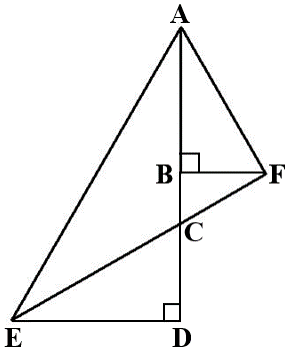# XAT 2015 Q10 : Area of right triangle

This aptitude question appeared as a part of the quantitative reasoning and data interpretation section of the XAT 2015. Question 10 of a total of 33 questions that appeared in this section in XAT 2015. This one is a medium level difficulty question and tests the following concepts: Similar triangles, trigonometric identities, and area of triangles.

## Question

In the diagram below, CD = BF = 10 units and ∠CED = ∠BAF = 30o. What would be the area of triangle AED? (Note: Diagram below may not be drawn to scale.)AD = AB + BC + CD = 10$$sqrt{3}\\$ + $\frac{10}{\sqrt3}\\$ + 10 #### Compute Area Area of triangle AED = $\frac{1}{2}\\$ ED×AD = $\frac{1}{2}\\$ × 10 $\sqrt{3}\\$ ×$10 + $$frac{10}{\sqrt3}\\$ + 10$\sqrt{3}\\$) = 50 ×$ $\sqrt{3}\\$ + 4)

The correct answer is Choice D.

## Online TANCET MBA CourseTry it Free!

Register in 2 easy steps and start learning in 5 minutes!